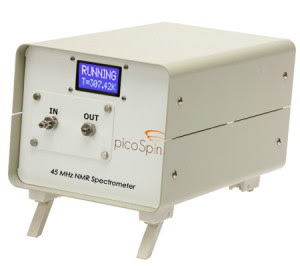## University of Ottawa NMR Facility Web Site

Please feel free to make suggestions for future posts by emailing Glenn Facey.

## Thursday, November 18, 2010

### The Phase of an NMR Spectrum

Most students know that the phase of an NMR spectrum has to do with the degree to which the NMR resonances are above or below the baseline of the spectrum (i.e. the amount of absorption and dispersion character). Most students have also learned that the phase of a periodic time domain function depends only on the value of the function at time zero. Thus, the only difference between a cosine and a sine function is where the function starts at time zero. A sine is said to be 90° out of phase with respect to a cosine. Many students do not understand the connection between the phase of their NMR spectrum and the phase of the periodic time domain function giving rise to their spectrum by way of a Fourier transform. The figure below is an attempt to make the connection. The left-hand portion of the figure shows equilibrium magnetization vectors being rotated by radio frequency pulses. 90° pulses along the x, y, -x and -y axes rotate the z magnetization vector to the -y, x, y and -x axes of the rotating frame, respectively according to the right-hand screw rule. After the pulse, the magnetization vector rotates in the rotating frame of reference at a frequency equal to the difference between the transmitter frequency and the frequency of the NMR resonance. In the figure, the magnetization is assumed to be rotating anti-clockwise representing an NMR resonance with a positive frequency with respect to the transmitter frequency. The NMR spectrometer measures the time dependent voltages on two of the four orthogonal axes of the rotating frame separated by 90° (quadrature detection). The time dependent voltages are proportional to the amount of magnetization on the axis as a function of time. One of the two time dependent voltages is called the "real" signal and the other is called the "imaginary" signal. Together these two signals make up the complex free induction decay (FID) which is Fourier transformed to produce the NMR spectrum. In the figure, the -y(t) voltage is the real FID and the x(t) voltage is the imaginary FID. The figure shows representations of the real and imaginary FIDs after the delivery of pulses along each of the orthogonal axes. Note that the phases of the real and imaginary FIDs depend on the pulse delivered. For example, after a 90°x pulse, the magnetization resides on the -y axis. The real FID (along the -y axis) starts at a maximum (cosine) and the imaginary FID (along the x axis) starts at zero (sine) and increases as the magnetization vector rotates anti-clockwise. The Fourier transform of the complex FID produces a real spectrum (typically the one displayed to the user) and an imaginary spectrum (typically not displayed to the user). Note that the degree of absorption vs. dispersion character in the spectrum depends on the phase of the FID signals. If an NMR spectrum is not in phase, perhaps due to a receiver dead time problem, it can be corrected after the collection of the data by calculating the phase angles needed to put the real spectrum enitirely in absorption mode and the imaginary spectrum entirely in dispersion mode.

## Wednesday, November 10, 2010

### New Bench Top 1H NMR Spectrometer

A very interesting product has just been put on the market from a company called picoSpin. - a 7 lb bench top 45 MHz 1H NMR spectrometer at a very tempting price. From the information available on their website (including a YouTube video), it looks as if this instrument would be an excellent teaching tool in a university laboratory.This is not meant as a product endorsement.